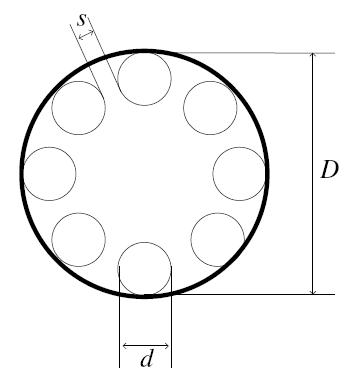2017-08-12 16:28

# Ball bearings

escription

The Swedish company SKF makes ball bearings. As explained by Britannica Online, a ball bearing is
"one of the two types of rolling, or anti friction, bearings (the other is the roller bearing).
Its function is to connect two machine members that move relative to one another so that the frictional resistance to motion is minimal. In many applications, one of the members is a rotating shaft and the other a fixed housing. Each ball bearing has three main parts: two grooved, ring like races and a number of balls. The balls fill the space between the two races and roll with negligible friction in the grooves. The balls may be loosely restrained and separated by means of a retainer or cage."

Presumably, the more balls you have inside the outer ring, the smoother the ride will be, but how many can you fit within the outer ring? You will be given the inner diameter of the outer ring, the diameter of the balls, and the minimum distance between neighboring balls. Your task is to compute the maximum number of balls that will fit on the inside of the outer ring (all balls must touch the outer ring).Input

The first line of input contains a positive integer n that indicates the number of test cases. Then follow n lines, each describing a test case. Each test case consists of three positive floating point numbers, D, d, s, where D is the inner diameter of the outer ring, d is the diameter of a ball, and s is the minimum distance between balls. All parameters are in the range [0.0001, 500.0].
Output

For each test case output a single integer m on a line by itself, where m is the maximum number of balls that can fit in the ball bearing, given the above constraints. There will always be room for at least three balls.
Sample Input

2
20 1 0.1
100.0 13.0 0.2
Sample Output

54
20

• 写回答
• 好问题 提建议
• 追加酬金
• 关注问题
• 邀请回答